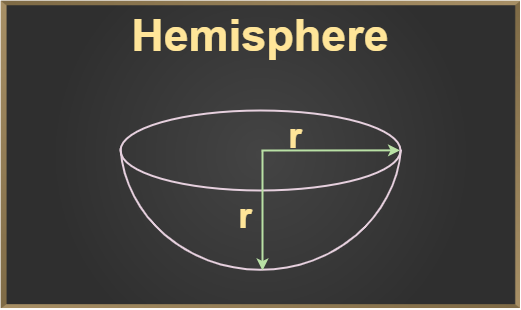GeeksforGeeks App
Open AppBrowser
Continue

## Related Articles

Mensuration is the branch of mathematics that deals with different geometric figures and calculating their parameters like length, breadth, radius, volume, surface area, lateral surface area, etc. The volume of a shape is defined as how much capacity a shape has or we can say how much material was required to form that shape.

## What is Hemisphere?

Hemisphere can be defined as a 3-dimensional shape that is formed by cutting a sphere into two equal halves. It is a combination of a half-spherical curve and a plane circular region.## What is the Volume of a Hemisphere?

A hemisphere is a three-dimensional object. It is half of a sphere. If a sphere is cut by a plane passing through its center, two hemispheres are obtained. A hemisphere has a flat circular base. The volume of a hemisphere is defined as the number of unit cubes it can hold.

## Volume of Hemisphere Formula

Hemisphere is just half of a sphere so its volume will also be just half.

As we know the volume of a sphere is 4/3πr3

Volume of Hemisphere = Volume of sphere/2

= (4/3πr3)/2

= 2/3πr3

It has been experimentally proved that the volume of a sphere is 2/3 of the volume of a cylinder with the same radius, and height equal to the diameter.

Volume of a cylinder with radius r and height as 2r = πr2(2r)

= 2πr3

So, the volume of the sphere will be = 2/3 × (2πr3)

= 4/3πr3

And similarly, the volume of the hemisphere can also be derived by dividing the volume of the sphere by 2.

Hence,

Volume of Hemisphere = 2/3πr3

where,
r is the radius of Hemisphere

## How to Find the Volume of a Hemisphere?

Volume of a hemisphere is calculated using the formula, Volume = 2πr3/3.

Use the following steps for finding the volume of a hemisphere.

Example: Find the volume of the hemisphere with a radius of 14 cm

Solution:

Step 1: Find the radius of a hemisphere, radius (r) = 14 cm
Step 2: Put the value of the radius in the given formula, Volume of hemisphere = 2πr3/3
Step 3: Solving the formula for volume,

Volume of hemisphere = 2πr3/3
= (2 × 3.14 × 143)/3
= 5744.10667 cm3

## Hemisphere Equation

Suppose, the radius of the is ‘r‘ units and its center is at origin (0,0,0) then its equation is given by,

x2 + y2 + z2 = r2

Cartesian form or equation of a hemisphere with the radius “r” at the center point (x1, y1, z1) is,

(x-x1)2 + (y- y1)2 + (z- z1)2 = r2

Spherical Coordinates of the hemisphere are,

x = r cos θ sin ∅
y = r sin θ cos ∅
z = r cos ∅

## Solved Examples on Volume of Hemisphere

Example 1: If the radius of a hemisphere is 21 cm. Find the volume of the hemisphere.

Solution:

We know that volume of hemisphere = 2/3 π r3

= 2/3 x 22/7 x 21 x 21 x 21

= 2 x 22 x 21 x 21

= 19404 cm3

Example 2: If the volume of the hemisphere is 30 cubic meters. It is melted and used to form hemispheres with a volume of 10 cubic meters. How many such hemispheres can be made?

Solution:

Certain number of hemispheres are made using a single big hemisphere.

Volume of small hemispheres = Volume of large hemisphere

Let there be n number of small hemispheres .

Then, n x volume of small hemisphere = volume of large hemisphere

n x 10 = 30

n = 30/10

n = 3

So, three hemispheres can be formed by melting the bigger hemisphere.

Example 3: Find the volume of a hemisphere of diameter 5 cm.

Solution:

volume of hemisphere = 2/3 π r3

Volume = 2/3 π (5/2)3

= 32.724 cm3

Example 4: If a hemisphere of radius 2 cm is fitted inside a cuboid and then water is filled inside the cuboid. Find the amount of water present in the cuboid.

Solution:

length of cuboid = 2r = 4 cm

breadth of cuboid = 2r = 4 cm

height of cuboid = r = 2 cm

volume of cuboid = lbh = 2 x 4 x 4

= 32 cm3

volume of hemisphere = 2/3 π r3

= 2/3 x (3.14) x (2)3

= 16.75 cm3

volume of water = volume of cuboid – volume of hemisphere

= 32 – 16.75

= 15.25 cm3

So, amount of water present in cuboid is 15.25 cm3

Example 5: If the volume of the hemisphere is 2.095 m3. Find the radius of the hemisphere.

Solution:

Volume of hemisphere = 2/3 π r3

2.095 = 2/3 π r3

2.095 = 2.095 r3

r3 = 1

r = 1 m

So, radius of hemisphere is 1 m.

## FAQs on Volume of Hemisphere

Question 1: What is a Hemisphere?

A hemisphere is a 3-D shape, it is half of a sphere. Cutting the sphere into two parts results in the creation of two hemispheres.

Question 2: If a sphere and a hemisphere have the same radii then what is the ratio of their volume?

If a sphere (volume V1) and a hemisphere(volume V2) have the same radii then the ratio of their volume is given by

V1 : V2 =  (4/3πr3) : (2/3πr3)

= 2 : 1

Question 3: How do we measure the volume of the hemisphere?

The volume of the hemisphere is measured in m3, cm3, liters, etc. The m3 is the standard unit of measurement. We can be converted mto liters according to our requirement.

Question 4: Write the formula for the surface area of the hemisphere.